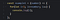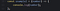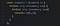# Calculating Time Complexity: Big-O Notation

Big-O is a notation (way of representing) that is used to approximately determine the efficiency or time complexity of a program.

The purpose of writing any program is solving a particular problem and Big-O is simply a measure of how well that problem is solved.

# Why Big-O is important?

In my previous article Simplifying Data structures, I talked about how we store our data impacts the performance of our program. More importantly, different operations like insertion, deletion, traversing, etc on different data structures take a different amount of time i.e. have different time complexity. So Big-O simply forms the foundation to decide which data structure to use for a particular problem.

Big-O notation can be easily understood by analyzing the graph between the size of the input given to the program(elements) corresponding to the number of operations that are performed. (will be clear in the example below but first)

## Assumptions —

1. Big-O is not calculated in terms of seconds or milliseconds, but a general term unit is used instead, and
2. Each operation consumes 1 unit of time.

Let's take an example for understanding the above. (I will be using javascript for demonstration purposes but the same is valid in every language.)

The function given below receives a number as a parameter and prints all the numbers from 1 up to that number.Prints up to a number

Now suppose If the

• Input — 5 (number).
• Output — 1 2 3 4 5

which implies that for an input 5(size of the input) the console.log statement gets executed 5 times (number of operations). and according to Big-O, each operation consumes 1 unit of time. So this function will take 5 units of time.

Similarly, if the input is 100(size of the input) the console.log statement will be executed 100 times and will take 100 units of time. General, If the input is n then it will take n units of time.

Now, if we plot this result on a graph, The graph will be linear, So the Big-O of this function is Linear and it is represented as O(n).

Let's take another example-

The function given below receives a number as a parameter and simply prints that number to the console.Prints the given number

Now suppose If the

• Input — 5 (number).
• Output — 5

which implies that for an input 5(size of the input) the console.log statement gets executed 1 time (number of operations) and takes 1 unit of time. Also, even if the input is 1 million (1000000), then too console.log statement will be executed only 1 time. So, no matter what the input is the function will always take a definite amount of time i.e 1 unit.

Now, Plotting this result on a graph, The graph will be constant, So the Big-O of this function is Constant and it is represented as O(1).

Taking it a step further,

The function given below receives a number as a parameter and logs all possible pairs of numbers.Log all possible pairs of numbers

Now Suppose if the,

• Input — 2 (number).
• Output — (1,1) (1,2) (2,1) (2,2), Also if
• Input — 3(number).
• Output — (1,1) (1,2) (1,3) (2,1) (2,2) (2,3),(3,1) (3,2), (3,3).

which implies that for an input 2(size of the input), the number of operations that takes place is 4 and takes 4 units of time. Also, if the input is 3, then the number of operations that takes place is 9 and takes 9 units of time.

Now, if we plot this on a graph, The graph will be quadratic, So the Big-O of this function is Quadratic and it is represented as O(n²).

Important Big-O Notations

• O(1)Constant
• O(n) Linear
• O(n²) Quadratic
• O(log n) Logarithmic
• O(n log n)Log-linear
• O(2^n) Exponential
• O(n!)Factorial

All these important notations and rules for determining Big-O will be discussed in the upcoming articles.

Web Developer